# CBSE Question Paper 2011 Class 12 Chemistry## myCBSEguide App

CBSE, NCERT, JEE Main, NEET-UG, NDA, Exam Papers, Question Bank, NCERT Solutions, Exemplars, Revision Notes, Free Videos, MCQ Tests & more.

CBSE Question Paper 2011 class 12 Chemistry conducted by Central Board of Secondary Education, New Delhi in the month of March 2011. CBSE previous year question papers with the solution are available in the myCBSEguide mobile app and website. The Best CBSE App for students and teachers is myCBSEguide which provides complete study material and practice papers to CBSE schools in India and abroad.

CBSE Question Paper 2011 class 12 Chemistry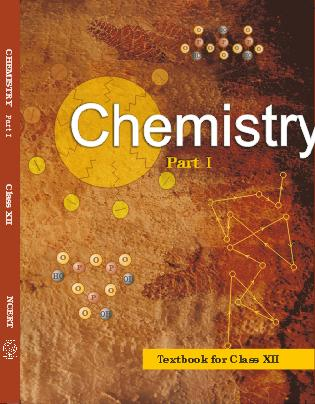## Class 12 Chemistry list of chapters

1. The Solid State
2. Solutions
3. Electrochemistry
4. Chemical Kinetics
5. Surface Chemistry
6. General Principles and Processes of Isolation of Elements
7. The p-Block Elements
8. The d and f Block Elements
9. Coordination Compounds
10. Haloalkanes and Haloarenes
11. Alcohols, Phenols and Ethers
12. Aldehydes, Ketones and Carboxylic Acids
13. Amines
14. Biomolecules
15. Polymers
16. Chemistry in Everyday life

## CBSE Question Paper 2011 class 12 Chemistry

General Instructions:

1. All questions are compulsory.
2. Marks for each question are indicated against it.
3. Question numbers 1 to 8 are very short-answer questions, carrying 1 mark each. Answer these in one word or about one sentence each.
4. Question numbers 9 to 18 are short-answer questions, carrying 2 marks each. Answer these in about 30 words each.
5. Question numbers 19 to 27 are short-answer questions of 3 marks each. Answer these in about 40 words each.
6. Question numbers 28 to 30 are long-answer questions of 5 marks each. Answer these in about 70 words each.
7. Use Log Tables, if necessary Use of calculators is not permitted.

1. ‘ Crystalline solids are anisotropic in nature.’ What does this statement mean? 

2. Express the relation between conductivity and molar conductivity of a solution held in a cell. 

3. Define ‘electrophoresis’. 

4. Draw the structure of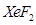molecule. 

5. Write the IUPAC name of the following compound: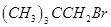6. Draw the structure of 3-methy lbutanal. 

7. Arrange the following compounds in an increasing order of their solubility in water: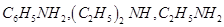8. What are biodegradable polymers? 

9. The chemistry of corrosion of iron is essentially an electrochemical phenomenon. Explain the reactions occurring during the corrosion of iron in the atmosphere. 

10. Determine the values of equilibrium constant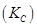and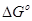for the following reaction: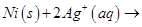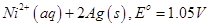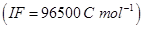11. Distinguish between ‘ rate expression’ and ‘ rate constant’ of a reaction. 

12. State reasons for each of the following: 

(i) The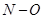bond in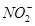is shorter than thebond in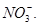(ii)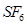is kinetically an inert substance.

OR

State reasons for each of the following:

(i) All the P-Cl bonds in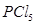molecule are not equivalent.

(ii) Sulphur has greater tendency for catenation than oxygen.

13. Assign reasons for the following: 

(i) Copper (I) ion is not known in aqueous solution.

(ii) Actinoids exhibit greater range of oxidation states than lanthanoids.

Ans. i) Because copper(I) ion is unstable in aqueous solution and undergoes, disproportionation.

ii) Because of comparable energies of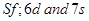orbitals in actinoids.

14. Explain the following giving one example for each: 

(i) Reimer -Tiemann reaction.

(ii) Friedel Craft’ s acety lation of anisole.

15. How would you obtain 

(i) Picric acid (2, 4, 6-trinitrophenol) from phenol,

(ii) 2-Methy lpropene from 2-methy lpropanol?

16. What is essentially the difference between a-form of glucose and b-form of glucose? Explain. 

17. Describe what you understand by primary structure and secondary structure of proteins. 

18. Mention two important uses of each of the following: 

(i) Bakelite

(ii) Nylon6

19. Silver crystallizes in face-centered cubic unit cell. Each side of this unit cell has a length of 400 pm. Calculate the radius of the silver atom. (Assume the atoms just touch each other on the diagonal across the face of the unit cell. That is each face atom is touching the four comer atoms.) 

20. Nitrogen pentoxide decomposes according to equation: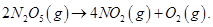This first order reaction was allowed to proceed at 40 °C and the data below were collected: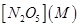Time (min) 0.400 0.00 0.289 20.0 0.209 40.0 0.151 60.0 0.109 80.0

(a) Calculate the rate constant. Include units with y our answer.

(b) What will be the concentration of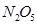after 100 minutes?

(c) Calculate the initial rate of reaction. 

21. Explain how the phenomenon of adsorption finds application in each of the following processes: 

(i) Product ion of vacuum

(ii) Heterogeneous catalysis

(iii) Froth Floatation process

OR

Define each of the following terms: 

(i) Micelles

(ii) Peptization

(iii) Desorption

22. Describe the principle behind each of the following processes: 

(i) Vapour phase refining of a metal.

(ii) Elect rolytic refining of a metal.

(iii) Recovery of silver after silver ore was leached with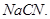23. Complete the following chemical equations: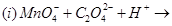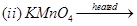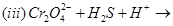24. Write the name, stereochemistry and magnetic behaviour of the following: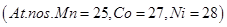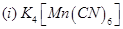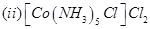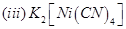(i) Haloalkanes easily dissolve in organic solvents, why?

(ii) What is known as a racemic mixture? Give an example.

(iii) Of the two bromoderivatives,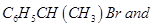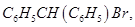which one is more reactive in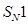substitution reaction and why?

26. (a) Explain why an alkylamine is more basic than ammonia.

(b) How would you convert

(i) Aniline to nitrobenzene

(ii) Aniline to iodobenzene? 

27. Describe the following giving one example for each: 

(i) Detergents

(ii) Food preservatives

(iii) Antacids

28. (a) Differentiate between molarity and molality for a solution. How does a change in temperature influence their values?

(b) Calculate the freezing point of an aqueous solution containing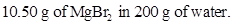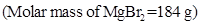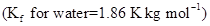OR

(a) Define the terms osmosis and osmotic pressure. Is the osmotic pressure of a solution a colliative property? Explain.

(b) Calculate the boiling point of a solution prepared by adding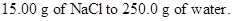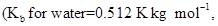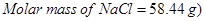29. (a) Give chemical tests to di stinguish between

(i) Propanal and propanone,

(ii) Benzaldehyde and acetophenone.

(b) How would you obtain

(i) But-2-enal from ethanal,

(ii) Butanoic acid from butanol,

(iii) Benzoic acid from ethylbenzene? 

OR

(a) Describe the following giving linked chemical equations:

(i) Cannizzaro reaction

(ii) Decarboxylation

(b) Complete the following chemical equations: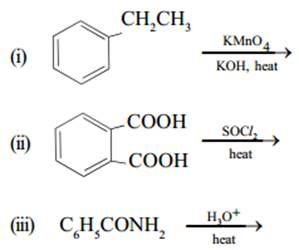30. (a) Explain the following:

(i)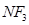is an exothermic compound whereas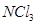is not.

(ii)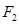is most reactive of all the four common halogens.

(b) Complete the following chemical equations: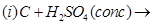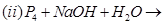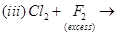OR

(a) Account for the following:

(i) The acidic strength decreases in the order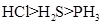(ii) Tendency to form pentahalides decreases down the group in group 15 of the periodic table.

(b) Complete the following chemical equations: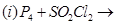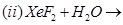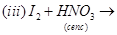These are questions only. To view and download complete question paper with solution install myCBSEguide App from google play store or login to our student dashboard.

## Chemistry Question Paper 2011

Download class 12 Chemistry question paper with solution from best CBSE App the myCBSEguide. CBSE class 12 Chemistry question paper 2011 in PDF format with solution will help you to understand the latest question paper pattern and marking scheme of the CBSE board examination. You will get to know the difficulty level of the question paper. CBSE question papers 2011 for class 12 Chemistry have 30 questions with solution.

## Previous Year Question Paper for class 12 in PDF

CBSE question papers 2018, 2017, 2016, 2015, 2014, 2013, 2012, 2011, 2010, 209, 2008, 2007, 2006, 2005 and so on for all the subjects are available under this download link. Practicing real question paper certainly helps students to get confidence and improve performance in weak areas.

To download CBSE Question Paper 2011 class 12 Accountancy, Chemistry, Physics, History, Political Science, Economics, Geography, Computer Science, Home Science, Accountancy, Business Studies and Home Science; do check myCBSEguide app or website. myCBSEguide provides sample papers with solution, test papers for chapter-wise practice, NCERT solutions, NCERT Exemplar solutions, quick revision notes for ready reference, CBSE guess papers and CBSE important question papers. Sample Paper all are made available through the best app for CBSE students and myCBSEguide website.